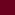(function(h,g,b,f,d,e,w){/*! Jssor Javascript Library */ \$Jssor\$=h.\$Jssor\$=h.\$Jssor\$||{};new(function(){});var p=function(){var b=this,a={};b.Pb=b.addEventListener=function(b,c){if(typeof c!="function")return;if(!a[b])a[b]=[];a[b].push(c)};b.removeEventListener=function(e,d){var b=a[e];if(typeof d!="function")return;else if(!b)return;for(var c=0;c<b.length;c++)if(d==b[c]){b.splice(c,1);return}};b.W=function(e){var c=a[e],d=[];if(!c)return;for(var b=1;b<arguments.length;b++)d.push(arguments[b]);for(var b=0;b<c.length;b++)try{c[b].apply(h,d)}catch(f){}}},j;(function(){j=function(a,b){this.x=typeof a=="number"?a:0;this.y=typeof b=="number"?b:0};})();var l={ye:function(a){return(~a&3)+(a&12)},ve:function(a){return(~a&12)+(a&3)},we:function(a){return(a&3)==1},Qe:function(a){return(a&3)==2},Ke:function(a){return(a&12)==4},Le:function(a){return(a&12)==8},Tc:function(a){return(a&3)>0},Zc:function(a){return(a&12)>0}},u={ee:37,ge:39},q,o={qe:0,pe:1,je:2,me:3,le:4,Zd:5},v=1,z=2,A=4,C=5,n,a=new function(){var c=this,p=o.qe,l=0,t=0,W=navigator.appName,s=navigator.userAgent;function L(){if(!p&&W=="Microsoft Internet Explorer"&&!!h.attachEvent&&!!h.ActiveXObject){var a=s.indexOf("MSIE");p=o.pe;t=parseFloat(s.substring(a+5,s.indexOf(";",a)));l=g.documentMode||t}}function O(){var a=/(opera)(?:.*version|)[ \/]([\w.]+)/i.exec(s);if(a){p=o.Zd;l=parseFloat(a)}}function y(){if(!p&&W=="Netscape"&&!!h.addEventListener){var b=s.indexOf("Firefox"),a=s.indexOf("Safari"),c=s.indexOf("Chrome");if(b>=0){p=o.je;l=parseFloat(s.substring(b+8))}else if(a>=0){var d=s.substring(0,a).lastIndexOf("/");p=c>=0?o.le:o.me;l=parseFloat(s.substring(d+1,a))}}}function m(){L();return p==v}function R(){y();return p==z}function S(){y();return p==A}function db(){O();return p==C}function Z(){return m()&&l<9||S()&&l<2}var J;function I(a){!J&&r(["transform","WebkitTransform","msTransform","MozTransform","OTransform"],function(b){if(!c.Nd(a.style[b])){J=b;return d}});return J}function V(a){return Object.prototype.toString.call(a)}var F;function r(a,c){if(V(a)=="[object Array]"){for(var b=0;b<a.length;b++)if(c(a[b],b,a))break}else for(var d in a)if(c(a[d],d,a))break}function eb(){if(!F){F={};r(["Boolean","Number","String","Function","Array","Date","RegExp","Object"],function(a){F["[object "+a+"]"]=a.toLowerCase()})}return F}function u(a){return a==f?String(a):eb()[V(a)]||"object"}function M(b,a){setTimeout(b,a||0)}function E(d,c,b){var a=d||"";r(c,function(c){var b=c.exec(a);if(b){var d=a.substr(0,b.index),e=a.substr(b.lastIndex+1,a.length-(b.lastIndex+1));a=d+e}});a=b+(a.indexOf(" ")!=0?" ":"")+a;return a}c.Od=db;c.g=M;c.Y=function(a){if(c.Id(a))a=g.getElementById(a);return a};c.Nb=function(a){return a?a:h.event};c.Ic=function(a){a=c.Nb(a);var b=new j;if(a.type=="DOMMouseScroll"&&R()&&l<3){b.x=a.screenX;b.y=a.screenY}else if(typeof a.pageX=="number"){b.x=a.pageX;b.y=a.pageY}else if(typeof a.clientX=="number"){b.x=a.clientX+g.body.scrollLeft+g.documentElement.scrollLeft;b.y=a.clientY+g.body.scrollTop+g.documentElement.scrollTop}return b};c.Hb=function(d,a,j){if(j&&Z())a=b.round(a);if(m()){var g="filter",i=d.style[g]||"",k=new RegExp(/[\s]*alpha\([^\)]*\)/g),h=b.round(100*a),e="";if(h<100)e="alpha(opacity="+h+") ";var c=E(i,[k],f);if(t<9)c=e+c;d.style[g]=c}if(!m()||t>=9)d.style.opacity=a==1?"":a};c.Xd=function(b,a,d){var f=b.style.filter,h=new RegExp(/[\s]*progid:DXImageTransform\.Microsoft\.Matrix\([^\)]*\)/g),g="progid:DXImageTransform.Microsoft.Matrix(M11="+a+", M12="+a+", M21="+a+", M22="+a+", SizingMethod='auto expand')",e=E(f,[h],g);b.style.filter=e;c.cd(b,d.y);c.Yc(b,d.x)};c.Sd=function(d,a){var h=a.t||0,f=a.zb==0?0:a.zb||1;if(m()&&t<9){var g=c.Rd(h/180*b.PI,f,f);c.Xd(d,g,c.Ud(g,a.lb,a.rb))}else{var k="rotate("+h%360+"deg) scale("+f+")",e=I(d),j=d.style[e],l=new RegExp(/[\s]*rotate\(.*?\)/g),n=new RegExp(/[\s]*scale\(.*?\)/g),i=E(j,[l,n],k);if(e)d.style[e]=i}};c.h=function(a,d,e,b){a=c.Y(a);if(a.addEventListener){d=="mousewheel"&&a.addEventListener("DOMMouseScroll",e,b);a.addEventListener(d,e,b)}else if(a.attachEvent){a.attachEvent("on"+d,e);b&&a.setCapture&&a.setCapture()}};c.Oe=function(a,d,e,b){a=c.Y(a);if(a.removeEventListener){d=="mousewheel"&&a.removeEventListener("DOMMouseScroll",e,b);a.removeEventListener(d,e,b)}else if(a.detachEvent){a.detachEvent("on"+d,e);b&&a.releaseCapture&&a.releaseCapture()}};c.Je=function(b,a){c.h(m()&&l<9?g:h,"mouseup",b,a)};c.jb=function(a){a=c.Nb(a);a.preventDefault&&a.preventDefault();a.cancel=d;a.returnValue=e};c.E=function(d,c){for(var b=[],a=2;a<arguments.length;a++)b.push(arguments[a]);return function(){for(var e=b.concat([]),a=0;a<arguments.length;a++)e.push(arguments[a]);return c.apply(d,e)}};c.Re=function(a,c){var b=g.createTextNode(c);a.innerHTML="";a.appendChild(b)};c.wc=function(a){a.innerHTML=""};c.Cc=function(c){for(var b=[],a=c.firstChild;a;a=a.nextSibling)a.nodeType==1&&b.push(a);return b};function H(a,c,b,e){if(!b)b="u";for(a=a?a.firstChild:f;a;a=a.nextSibling)if(a.nodeType==1){if(a.getAttribute(b)==c)return a;if(e){var d=H(a,c,b,e);if(d)return d}}}c.F=H;function X(a,d,b,g){if(!b)b="u";var c=[];for(a=a?a.firstChild:f;a;a=a.nextSibling)if(a.nodeType==1){a.getAttribute(b)==d&&c.push(a);if(g){var e=H(a,d,b,g);if(e.length)c=c.concat(e)}}return c}c.se=X;function N(a,c,d){for(a=a?a.firstChild:f;a;a=a.nextSibling)if(a.nodeType==1){if(a.tagName==c)return a;if(d){var b=N(a,c,d);if(b)return b}}}c.re=N;function P(a,e,g){var b=[];for(a=a?a.firstChild:f;a;a=a.nextSibling)if(a.nodeType==1){a.tagName==e&&b.push(a);if(g){var c=P(a,e,d);if(c.length)b=b.concat(c)}}return b}c.ue=P;c.te=function(b,a){return b.getElementsByTagName(a)};c.m=function(c){for(var b=1;b<arguments.length;b++){var a=arguments[b];if(a)for(var d in a)c[d]=a[d]}return c};c.Nd=function(a){return u(a)=="undefined"};c.Ac=function(a){return u(a)=="function"};c.Fe=Array.isArray||function(a){return u(a)=="array"};c.Id=function(a){return u(a)=="string"};c.Zb=function(a){return!isNaN(parseFloat(a))&&isFinite(a)};c.f=r;c.hc=function(a){return c.ad("DIV",a)};c.He=function(a){return c.ad("SPAN",a)};c.ad=function(b,a){a=a||g;return a.createElement(b)};c.Q=function(){};c.Ge=function(b,c,a){b.setAttribute(c,a)};c.oc=function(a){return a.className};c.lc=function(b,a){b.className=a?a:""};c.Be=function(b,a){b.style.cursor=a};c.ob=function(b,a){b.style.display=a};c.X=function(b,a){b.style.overflow=a};c.tc=function(a){return a.parentNode};c.hb=function(a){c.ob(a,"none")};c.w=function(a,b){c.ob(a,b==e?"none":"")};c.N=function(b,a){b.style.position=a};c.id=function(a){return parseInt(a.style.top,10)};c.fb=function(a,b){a.style.top=b+"px"};c.fd=function(a){return parseInt(a.style.left,10)};c.S=function(a,b){a.style.left=b+"px"};c.dc=function(a){return parseInt(a.style.width,10)};c.bc=function(c,a){c.style.width=b.max(a,0)+"px"};c.ac=function(a){return parseInt(a.style.height,10)};c.Yb=function(c,a){c.style.height=b.max(a,0)+"px"};c.Hd=function(a){return a.style.cssText};c.Ed=function(b,a){b.style.cssText=a};c.Yc=function(b,a){b.style.marginLeft=a+"px"};c.cd=function(b,a){b.style.marginTop=a+"px"};c.Sc=function(b,a){b.style.backgroundColor=a};c.yd=function(c,a){c.style.clip="rect("+b.round(a.a)+"px "+b.round(a.l)+"px "+b.round(a.k)+"px "+b.round(a.b)+"px)"};c.s=function(){return+new Date};c.p=function(b,a){b.appendChild(a)};c.Mc=function(c,b,a){c.insertBefore(b,a)};c.Xb=function(b,a){b.removeChild(a)};c.Cd=function(b,a){r(a,function(a){c.Xb(b,a)})};c.Bd=function(a){c.Cd(a,c.Cc(a))};c.Kc=function(b,a){var c=g.body;while(a&&b!=a&&c!=a)try{a=a.parentNode}catch(d){return e}return b==a};c.z=function(b,a){return b.cloneNode(a)};function K(a,b,c){b.onload=f;a&&a(b,c)}c.yb=function(g,e){var b=new Image;b.onload=a.E(f,K,e,b);b.onabort=a.E(f,K,e,b,d);b.src=g;c.Od()&&l<11.6&&K(e,b)};c.nd=function(c,k,j,i){if(i)c=a.z(c,d);for(var h=a.te(c,k),f=h.length-1;f>-1;f--){var b=h[f],e=a.z(j,d);a.lc(e,a.oc(b));a.Ed(e,a.Hd(b));var g=a.tc(b);a.Mc(g,e,b);a.Xb(g,b)}return c};var x;function gb(b,n){var i=this,j,g,k,h;function f(){var c=j;if(g)c+="dn";else if(h)c+="hv";else if(k)c+="av";a.lc(b,c)}function p(){h=d;f()}function q(){h=e;f()}function o(){x.push(i);g=d;f()}i.pd=function(){g=e;f()};i.od=function(a){k=a;f()};b=c.Y(b);if(!x){c.Je(function(){var a=x;x=[];r(a,function(a){a.pd()})});x=[]}j=c.oc(b);a.h(b,"mousedown",o);if(n&&m()&&l<7){a.h(b,"mouseover",p);a.h(b,"mouseout",q)}}c.gd=function(b,a){return new gb(b,a)};var k;function D(){if(!k){k={n:c.Hb,a:c.fb,b:c.S,u:c.bc,y:c.Yb,xb:c.ob,c:c.yd,Hc:c.Yc,Gc:c.cd,wb:c.Sd,K:c.N};k.n=k.n;k.a=k.a;k.b=k.b;k.u=k.u;k.y=k.y;k.xb=k.xb;k.c=k.c}return k}function Q(){D();k.Hc=k.Hc;k.Gc=k.Gc;k.wb=k.wb;return k}c.C=function(c,b){var a=D();r(b,function(d,b){a[b]&&a[b](c,d)})};c.xd=function(b,a){Q();c.C(b,a)};q=new function(){var a=this;function b(d,g){for(var j=d.length,i=d.length,h=g.length,f=[],c=0;c<i;c++)for(var k=f[c]=[],b=0;b<h;b++){for(var e=0,a=0;a<j;a++)e+=d[c][a]*g[a][b];k[b]=e}return f}a.tb=function(d,c){var a=b(d,[[c.x],[c.y]]);return new j(a,a)}};c.Rd=function(d,a,c){var e=b.cos(d),f=b.sin(d);return[[e*a,-f*c],[f*a,e*c]]};c.Ud=function(d,c,a){var e=q.tb(d,new j(-c/2,-a/2)),f=q.tb(d,new j(c/2,-a/2)),g=q.tb(d,new j(c/2,a/2)),h=q.tb(d,new j(-c/2,a/2));return new j(b.min(b.min(b.min(e.x,f.x),g.x),h.x)+c/2,b.min(b.min(b.min(e.y,f.y),g.y),h.y)+a/2)};n=function(t,v,h,R,E,w){var f=this,p,m,n,x,y=0,C,P,O,J,k=0,z=0,G,l=t,u=t+v,j,g,o,A=[],B;function H(a){return a>=l&&a<=u}function K(a){j+=a;g+=a;l+=a;u+=a;r(A,function(b){b,b.Rc(a)})}function Q(a,b){var c=a-j+t*b;K(c);return g}function s(t){var p=t;if(o&&(p>=g||p<=j))p=((p-j)%o+o)%o+j;if(k!=p||!G||x){var m=b.min(p,g);m=b.max(m,j);if(H(m)||H(k)){if(w){var L=(m-l)/v,e={};for(var n in w){var S=P[n]||1,J=O[n]||[0,1],i=(L-J)/J;i=b.min(b.max(i,0),1);i=i*S;var F=b.floor(i);if(i!=F)i-=F;var Q=C[n]||C.L,I=Q(i),q,K=E[n],D=w[n];if(a.Zb(D))q=K+(D-K)*I;else{q=c.m({v:{}},E[n]);r(D.v,function(c,b){var a=c*I;q.v[b]=a;q[b]+=a})}e[n]=q}if(E.j)e.wb={t:e.t||0,zb:e.j,lb:h.lb,rb:h.rb};if(w.c&&h.sb){var s=e.c.v,y=(s.a||0)+(s.k||0),u=(s.b||0)+(s.l||0);e.b=(e.b||0)+u;e.a=(e.a||0)+y;e.c.b-=u;e.c.l-=u;e.c.a-=y;e.c.k-=y}r(e,function(b,a){B[a]&&B[a](R,b)})}f.Xc(z-l,m-l)}z=m;a.f(A,function(a){a.ab(m)});var N=k,M=t;k=t;G=d;f.Rb(N,M)}}function F(a,c){g=b.max(g,a.Vc(g*c,1));A.push(a)}function I(){if(p){var d=c.s(),e=b.min(d-y,80),a=k+e*n;y=d;if(a*n>=m*n)a=m;s(a);if(!x&&a*n>=m*n)L(J);else M(I,h.R)}}function q(a,e,h){if(!p){p=d;x=h;J=e;a=b.max(a,j);a=b.min(a,g);m=a;n=m<k?-1:1;f.jc();y=c.s();I()}}function L(a){if(p){x=p=e;f.ic();a&&a()}}f.vb=function(a,b,c){q(a?k+a:g,b,c)};f.wd=function(b,a,c){q(b,a,c)};f.vd=function(a,b){q(j,a,b)};f.ud=function(a,b){q(g,a,b)};f.P=function(){L()};f.rd=function(a){q(a)};f.td=function(){return k};f.sd=function(){return m};f.Eb=function(){return z};f.ab=s;f.Bb=function(){s(j)};f.zc=function(){s(g)};f.sb=function(a){s(k+a)};f.Dd=function(){return p};f.zd=function(a){o=a};f.Vc=Q;f.Rc=K;f.nc=function(a){F(a,0)};f.ed=function(a){F(a,1)};f.Rb=c.Q;f.jc=c.Q;f.ic=c.Q;f.Xc=c.Q;h=a.m({R:10},h);o=h.ld;B=c.m({},D(),h.sc);j=l=t;g=u=t+v;var P=h.o||{},O=h.I||{};C=a.m({L:a.Ac(h.d)&&h.d||i.Qb},h.d)}},i={hd:function(a){return a},Qb:function(a){return-b.cos(a*b.PI)/2+.5},T:function(a){return-a*(a-2)},qc:function(a){return(a*=2)<1?1/2*a*a:-1/2*(--a*(a-2)-1)},kd:function(a){return-1/2*(b.cos(b.PI*a)-1)},md:function(a){return a==0||a==1?a:(a*=2)<1?1/2*b.pow(2,10*(a-1)):1/2*(-b.pow(2,-10*--a)+2)},db:function(a){return 1-b.cos(a*b.PI*2)},dd:function(a){return b.sin(a*b.PI*2)},Ad:function(a){return 1-((a*=2)<1?(a=1-a)*a*a:(a-=1)*a*a)},nb:function(a){return(a*=2)<1?a*a*a:(a=2-a)*a*a}},m={rc:1,Fd:2,qd:3};function y(e,B){var c=this;p.call(c);e=a.Y(e);var r,y,x,q,k=0,h,l,g,u,v,j,i,o,n,A=[],w=[];function t(a){a!=-1&&w[a].od(a==k)}function z(a){c.W(m.rc,a*l)}c.ib=e;c.Jb=function(a){if(a!=q){var e=k,d=b.floor(a/l);k=d;q=a;t(e);t(d);c.W(m.Fd,a)}};c.Ab=function(b){a.w(e,b)};var s;c.cc=function(F){if(!s){r=b.ceil(F/l);k=0;var q=o+u,t=n+v,E=b.ceil(r/g)-1;y=o+q*(!j?E:g-1);x=n+t*(j?E:g-1);a.bc(e,y);a.Yb(e,x);for(var h=0;h<r;h++){var D=a.He();a.Re(D,h+1);var p=a.nd(i,"NumberTemplate",D,d);a.N(p,"absolute");var B=b.floor(h/g);a.S(p,!j?q*B:h%g*q);a.fb(p,j?t*B:h%g*t);a.p(e,p);A[h]=p;a.h(p,"click",a.E(f,z,h));w[h]=a.gd(p,d)}c.W(m.qd);s=d}};c.mb=h=a.m({Sb:0,Ub:0,Wb:1},B);i=a.F(e,"prototype");o=a.dc(i);n=a.ac(i);a.Xb(e,i);l=h.Dc||1;g=h.jd||1;u=h.Sb;v=h.Ub;j=h.Wb-1}function s(e,g,c,f){var b=this;n.call(b,0,0);a.dc(e);a.ac(e);}var r,k={},t;new function(){var I=1,H=2,J=4,G=8,N=256,O=512,M=1024,L=2048,z=L+I,y=L+H,E=O+I,C=O+H,D=N+J,A=N+G,B=M+J,F=M+G;function S(a){return(a&H)==H}function T(a){return(a&J)==J}k.mc=function(g){for(var e=g.e,f=g.i,k=g.cb,l=g.pb,d={},a=0,b=0,i=e-1,j=f-1,h=l-1,c,b=0;b<f;b++)for(a=0;a<e;a++){c=b+","+a;switch(k){case z:d[c]=h-(a*f+(j-b));break;case B:d[c]=h-(b*e+(i-a));break;case E:d[c]=h-(a*f+b);case D:d[c]=h-(b*e+a);break;case y:d[c]=a*f+b;break;case A:d[c]=b*e+(i-a);break;case C:d[c]=a*f+(j-b);break;default:d[c]=b*e+a}}return d};k.O=function(h){var m=h.e,n=h.i,e=h.cb,l=h.pb,j={},k=0,c=0,d=0,f=m-1,g=n-1,o=l-1,i;switch(e){case z:case C:case E:case y:var a=0,b=0;break;case A:case B:case D:case F:var a=f,b=0;break;default:e=F;var a=f,b=0}c=a;d=b;while(k<l){i=d+","+c;if(T(e)||S(e))j[i]=o-k++;else j[i]=k++;switch(e){case z:case C:c--;d++;break;case E:case y:c++;d--;break;case A:case B:c--;d--;break;case F:case D:default:c++;d++}if(c<0||d<0||c>f||d>g){switch(e){case z:case C:a++;break;case A:case B:case E:case y:b++;break;case F:case D:default:a--}if(a<0||b<0||a>f||b>g){switch(e){case z:case C:a=f;b++;break;case E:case y:b=g;a++;break;case A:case B:b=g;a--;break;case F:case D:default:a=0;b++}if(b>g)b=g;else if(b<0)b=0;else if(a>f)a=f;else if(a<0)a=0}d=b;c=a}}return j};k.De=function(d){for(var g={},e=[],a,c,f=0;f<d.i;f++)for(a=0;a<d.e;a++)e.push({Ae:f+","+a,pc:b.ceil(1e5*b.random())%13});e.sort(function(a,b){return a.pc-b.pc});for(var c=0;c<d.pb;c++)g[e[c].Ae]=c;return g};function Q(e){var f=0,h=e.A,d=[],c=h(e);if(e.ze){a.f(c,function(a){f=b.max(f,a)});a.f(c,function(a,b){c[b]=f-a})}a.f(c,function(b,c){var a=d[b];if(!a){d[b]=a=[];a.Bc=b}a.push(c)});var g=[].concat(d);g.sort(function(a,b){return a.Bc-b.Bc});return{xe:c,Se:g}}var K=[];function P(d){var c={R:40,q:200,g:0,e:1,i:1,n:0,j:0,c:0,sb:e,H:e,r:0,ze:e,A:k.De,cb:F,Mb:{vc:0,Ue:0},d:i.Qb,o:{},qb:[],I:{}};a.m(c,d);c.pb=c.e*c.i;if(a.Ac(c.d))c.d={L:c.d};c.xc=b.round(c.q/c.R);c.kc=R(c);c.Te=function(b,a){b/=c.e;a/=c.i;var f=b+"x"+a;if(!c.qb[f]){c.qb[f]={u:b,y:a};for(var d=0;d<c.e;d++)for(var e=0;e<c.i;e++)c.qb[f][e+","+d]={a:e*a,l:d*b+b,k:e*a+a,b:d*b}}return c.qb[f]};return c}function R(d){var c=d.d;if(!c.L)c.L=i.Qb;var e=d.xc,f=c.Z;if(!f){var g=a.m({},d.d,d.o);f=c.Z={};a.f(g,function(n,l){var g=c[l]||c.L,j=d.o[l]||1;if(!a.Fe(g.Z))g.Z=[];var h=g.Z[e]=g.Z[e]||[];if(!h[j]){h[j]=;for(var k=1;k<=e;k++){var i=k/e*j,m=b.floor(i);if(i!=m)i-=m;h[j][k]=g(i)}}f[l]=h})}return f}t=function(J,S,C,Y,g,t,r,bb,cb,O){g=K[g.B]=K[g.B]||P(g);var o=this,k=Y.eb,p=g.H,m,M,w,q,T={},s={},F={},i,h,D,j,A,x,z,y,E,G,H=f,X,v,u,B=d,n=e,U=e,R,I=g.Mb.vc||0,L=g.Mb.Ue||0,V=cb.Pe;function eb(){if(V){k=Y.Gb;g.j=0}k=a.z(k,d);a.w(k);a.S(k,0);j=g.Te(t,r);G=Q(g);for(w=0;w<g.i;w++)for(c=0;c<g.e;c++){m=w+","+c;var K=e,N=e;if(I&&c%2){if(l.Tc(I))K=!K;if(l.Zc(I))N=!N}if(L&&w%2){if(l.Tc(L))K=!K;if(l.Zc(L))N=!N}g.a=g.a||g.c&4;g.k=g.k||g.c&8;g.b=g.b||g.c&1;g.l=g.l||g.c&2;A=N?g.k:g.a;x=N?g.a:g.k;z=K?g.l:g.b;y=K?g.b:g.l;g.c=A||x||z||y;if(g.Ve)g.n=2;D={};h={a:0,b:0,n:1,u:t,y:r};i=a.m({},h);M=a.m({},j[m]);if(g.n)h.n=2-g.n;if(g.j==d)g.j=1;if(g.j||g.t){h.j=g.j?g.j-1:1;i.j=1;h.t=g.t?360:0;i.t=0}else{if(g.c){var O=g.We||1,n=M.v={};if(A&&x){n.a=j.y/2*O;n.k=-n.a}else if(A)n.k=-j.y*O;else if(x)n.a=j.y*O;if(z&&y){n.b=j.u/2*O;n.l=-n.b}else if(z)n.l=-j.u*O;else if(y)n.b=j.u*O}D.c=M;i.c=j[m]}if(g.r){var B=g.r;if(!K)B=l.ye(B);if(!N)B=l.ve(B);var ab=g.M||1,cb=g.U||1;if(l.we(B))h.b+=t*ab;else if(l.Qe(B))h.b-=t*ab;if(l.Ke(B))h.a+=r*cb;else if(l.Le(B))h.a-=r*cb}a.f(h,function(b,c){if(a.Zb(b))if(b!=i[c])D[c]=b-i[c]});T[m]=p?i:h;var Z=b.round(G.xe[m]*g.g/g.R);s[m]=new Array(Z);s[m].Ne=Z;var P=g.xc;for(E=0;E<=P;E++){var f={};a.f(D,function(e,c){var m=g.kc[c]||g.kc.L,l=m[g.o[c]||1],k=g.I[c]||[0,1],d=(E/P-k)/k*P;d=b.round(b.min(P,b.max(d,0)));var j=l[P-d];if(a.Zb(e))f[c]=i[c]+e*j;else{var h=f[c]=a.m({},i[c]);h.v=[];a.f(e.v,function(c,b){var a=c*j;h.v[b]=a;h[b]+=a})}});if(i.j)f.wb={t:f.t||0,zb:f.j,lb:t,rb:r};if(f.c&&g.sb){var R=f.c.v,W=(R.a||0)+(R.k||0),U=(R.b||0)+(R.l||0);f.b=(f.b||0)+U;f.a=(f.a||0)+W;f.c.b-=U;f.c.l-=U;f.c.a-=W;f.c.k-=W}f.xb="";s[m].push(f)}}var o=bb;if(C)if(V)o=C.Gb;else if(C.Td())o=C.eb;o=a.z(o,d);a.w(o);a.S(o,0);if(p)H=o;else{J.Ib(o);H=k}X=G.Se;a.f(X,function(c){if(c)for(var e=0;e<c.length;e++){var b=c[e];q=a.z(H,d);a.C(q,T[b]);a.X(q,"hidden");a.N(q,"absolute");S.Yd(q);F[b]=q;a.w(F[b],p)}});p&&J.Ib(k);v=a.s();u=1;a.f(s,function(a){u=b.max(a.length,u)});_FrameCurrent=0}function fb(){if(B&&!n){var c=b.ceil((a.s()-v)/g.R)-1;if(c>=u-1){!p&&J.Ib(k);S.kb();B=e}else a.f(s,!B||n?a.Q:function(e,f){var d=c;if(p){d=u-c-1;d=b.max(d,e.Ne)}d=b.min(d,e.length-1);e[d]&&a.xd(F[f],e[d])})}return B}function ab(c){function b(){if(!U)if(n||fb())a.g(b,c);else db()}a.g(b,c)}var W;function db(){if(!W){W=d;O&&O()}}o.P=function(){if(!R){R=d;v-=2e6;return d}};o.vb=function(){ab(g.R)};o.Vd=function(){eb()};var Z;o.Fb=function(){if(!n){n=d;Z=a.s()-v}};o.Wd=function(){U=d};o.Qd=function(){if(n){n=e;v=a.s()-Z}};o.yc=g};function x(l,Kb){function Ub(){var a=0;if(G)a=Ab%G.length;else a=b.floor(b.random()*G.length);Ab++;G[a].B=a;return G[a]}function Gb(e,d){var b=r>0?r:c.J;a.S(e,eb*d*(b&1));a.fb(e,fb*d*(b>>1&1))}function tb(b){V&&a.jb(b)}function Bb(){var c=this,b=a.hc();a.C(b,J);a.N(b,"absolute");c.ib=b;c.Ib=function(f){var c=a.z(f,d);a.wc(b);a.p(b,c)};c.Yd=function(c){a.p(b,c)};c.kb=function(){a.wc(b)}}function Yb(u,c,B){var b=this;n.call(b,-x,x+1,{});var h,m,g,l,j,r,i,k,p;function v(c){p=d;j&&a.hb(j);c&&c(b)}function w(){g.Dd();b.Kb()}function y(){}function t(){g=f;if(q.gc&1&&!(q.gc&4))b.Lb().zc();else h.Bb()}b.B=B;b.yb=function(c){if(b.eb)if(!p){var d=b.eb.src;j&&Wb&&a.w(j);return a.yb(d,a.E(f,v,c))}c&&c(b)};b.Td=function(){return p};b.Ld=function(b){a.f(r,function(c){a.h(c,"click",b)})};b.Jd=function(){a.hb(c)};b.Ob=function(){a.w(c)};b.Kb=function(){g&&g.P()};b.Lb=function(){if(!h||h.V!=q.V){h&&h.Bb();var b=a.se(c,"caption");h=new q.D(c,b,q,d);m=new q.D(c,b,q);h.zc();h.V=q.V=q.V||a.s();g=f}return h};b.Pd=function(){t()};b.Fc=function(d,c){c&&b.Ob();var a=g||b.Lb();a.vd(d);g=a};b.Md=function(a){if(q.uc&1&&!(q.uc&4)){m.Bb();m.ud(a);g=m}else a&&a()};b.ne=function(){b.Kb();t();b.Ob()};b.Xc=function(c,b){var a=x-b;Gb(l,a)};var s=a.F(c,"thumb");if(s){a.z(s,d);a.hb(s)}a.w(c);if(z)j=a.z(z,d);r=a.ue(c,"A",d);i=a.F(c,"image");if(i){if(i.tagName=="A"){k=a.z(i,e);a.h(k,"click",tb);a.C(k,J);a.ob(k,"block");a.Hb(k,0);a.Sc(k,"#000");i=a.re(i,"IMG");i.border=0}a.C(i,J)}b.eb=i;b.ie=k;b.Gb=c;b.gb=l=Z();a.p(l,c);j&&a.p(l,j);b.yb(a.Q);b.Lb();u.Pb(1,y);u.Pb(2,w)}function Xb(){var q=this;p.call(q);var o=Z(),m=[],l,s,K,ib="mousedown",G="mousemove",ob="mouseup",U="mouseup";p.call(q);function Pb(b){if(!P)Ob(b);else!L&&a.jb(b)}var t,J,ub;function Ob(b){var h=y;a.s();J=w;ub=v.sd();v.P();if(!J)r=0;V=e;y=d;if(l){C();l.Fb();D=d}if(L){var f=b.touches;mb=f.clientX;nb=f.clientY}else{var c=a.Ic(b);mb=c.x;nb=c.y;a.jb(b)}H=0;t=T.Eb();!h&&a.h(g,G,Ib);q.W(2)}function Ib(h){var d;if(L){var g=h.touches;if(g&&g.length>0)d=new j(g.clientX,g.clientY)}else d=a.Ic(h);if(d){var e=d.x-mb,f=d.y-nb;if(!r){if(c.x==3)if(b.abs(e)>=b.abs(f))r=1;else r=2;else{r=c.x;if(L&&(b.abs(f)-b.abs(e))*(r==1?1:-1)>3)r=-1}if(b.floor(t)!=t)r=c.J}if(r==1){K=t-e/eb;H=e}else{K=t-f/fb;H=f}if(r>0){a.jb(h);if(!w)v.Ce(t);else v.de(K)}}}function Kb(){if(y){a.s();a.Oe(g,G,Ib);V=H;v.P();if(!V&&J)v.rd(ub);else{var h=k;if(h==-1)h=0;var f=T.td(),d=h;if(!H)d=f;else if(b.abs(H)>=c.Tb){d=b.floor(f);if(H<0)d+=1}v.Uc(f,d,b.abs(d-f)*pb)}y=e}}function vb(a){wb=k;k=F(a);Eb=m[k];Hb(k);return k}function jb(h,n,k){if(yb){v.P();B.Wc();var d=k;if(!d)d=pb;var l=T.bd();if(l.K||!cb(h)){var i=F(h),j=a.E(f,Lb,i,m[i],n),c=T.Eb(),e=h,g=c==e?0:d*b.abs(e-c);g=b.min(g,pb*x*1.5);v.Uc(c,e,g,j)}}}function R(a){if(hb)if(!Db){Db=d;bb=0}else bb=a||Sb;else bb=6e8}function Xb(b,c){r=0;var a=k;if(cb(b)){if(l){C(Rb(b)&&D&&l.yc.G);l.Qd()}}else{vb(b);B.kb()}E=d;M=0;D=e;B.Wc();if(!cb(a))m[a]&&m[a].ne();c.Fc(function(){R();E=e});q.W(1,a,k)}function Lb(c,b,a){a&&a()}function Hb(b,c){a.f(Q,function(a){a.Jb(F(b),c)})}function Ab(){E=e;if(W){D=e;M=1;B.ae(F(k+1))}else{var a=k;if(a==-1)a=0;else a+=Cb;jb(a)}}function Mb(){E=d;if(k==-1)Ab();else m[k].Md(Ab,W)}function sb(){if(S&&hb&&!y&&!w&&!E&&!P&&(!M||D)||rb){bb-=60;if(bb<0&&(!Fb||Y)){rb=e;Mb()}}a.g(sb,60)}function Wb(f){var e=this,g=f.length;n.call(e,0,0,{ld:g});e.pb=g;e.bd=function(){var a=e.Eb(),g=b.floor(a),d=f[g].B,c=a-b.floor(a);return{B:d,K:c}};e.Rb=function(e,a){var c=b.floor(a);if(c!=a&&a>e)c++;Hb(c,d)};a.f(f,function(a){a.zd(g);e.ed(a);a.Rc(zb/(c.J==1?eb:fb))})}function Tb(){var c=this,p=new Bb,h=new Bb,g=Z();a.X(g,"");var b;n.call(c,-1,2,{d:i.hd,sc:{K:Gb}},g,{K:1},{K:-1});function r(a){if(S&&hb&&!y&&!w&&!P){k=F(a);l.vb()}}function q(b,a){C();E=d;D=e;M=0;j();a.Fc(function(){R();E=e},d)}function j(){if(!s){s=Z();a.Sc(s,"#000");a.Hb(s,0);a.p(o,s)}var c=b&&b.ie,d=Jb<2||!M||w||y||!c;a.Bd(s);if(!d)a.p(s,c);a.w(s,M==1||D)}c.gb=g;c.ae=function(d){b=m[d];b.Pd();var e=d;c.Vc(e,1);c.ab(e);var i=a.E(f,q,d,b),g=Ub();l=new kb(p,h,Eb,b,g,gb,qb,z,N,i);l.Vd();C(g.G);b.Jd();v.Jc(e);vb(d);j();b.yb(a.E(f,r,d,b))};c.Vb=function(){if(l){C();l.Fb()}};c.kb=function(){if(l){l.Wd();h.kb();b.Ob()}};c.Wc=j;C();a.p(g,p.ib);a.p(g,h.ib)}function Nb(l,o){var a=this,g,h,i,b,j,c;n.call(a,-1e8,2e8);a.jc=function(){w=d};a.ic=function(){w=e;j=e;var a=l.bd();a.K==0&&Xb(a.B,m[a.B])};a.Rb=function(e,d){var a;if(j)a=c;else{a=h;if(i)a=d/i*(h-g)+g}b.ab(a)};a.Uc=function(c,d,b,e){m[k]&&m[k].Kb();g=c;h=d;i=b;a.ab(0);a.wd(b,e)};a.Ce=function(b){j=d;c=b;a.vb(b,f,d)};a.de=function(a){c=a};a.Jc=function(a){!w&&b.ab(a)};b=new n(-1e8,2e8);b.nc(l);b.nc(o)}q.be=function(a,c,b){if(!y&&!cb(a)){if(l){C();l.Fb()}jb(a,c,b)}};q.Vb=function(){B.Vb()};q.Oc=function(){R(1)};q.he=m;B=new Tb;a.p(O,B.gb);q.ib=o;if(c.J==1)a.bc(o,eb*x-X);else a.Yb(o,fb*x-X);a.X(o,"hidden");for(var I=0;I<ab.length;I++){var Qb=ab[I],lb=new Yb(q,Qb,I);lb.Ld(tb);a.p(o,lb.gb);m.push(lb)}T=new Wb(m);v=new Nb(T,B);v.Jc(0);a.Be(o,"move");a.p(A,o);if(Vb){if(h.navigator.msPointerEnabled){L=d;ib="MSPointerDown";G="MSPointerMove";ob="MSPointerUp";U="MSPointerCancel";if(c.x){var db="none";if(c.x==1)db="pan-y";else if(c.x==2)db="pan-x";a.Ge(o.style,"-ms-touch-action",db)}}else if("ontouchstart"in h||"createTouch"in g){L=d;ib="touchstart";G="touchmove";ob="touchend";U="touchcancel"}a.h(o,ib,Pb);a.h(g,ob,Kb);a.h(g,U,Kb)}hb=d;if(W)sb();else a.h(h,"load",sb)}var o=this,l=a.Y(l),c=a.m({Pc:e,Lc:e,Nc:1,Qc:3e3,Db:400,Tb:20,Cb:0,bb:1,ub:0,Ec:1,J:1,x:1},Kb),N=c.fe,q=a.m({D:s,gc:1,uc:1},c.Ye),lb=c.ke,ib=c.Kd,jb=c.Xe,db=c.Ec,A=a.F(l,"slides",f,db),z=a.F(l,"loading",f,db),vb=a.F(l,"navigator",f,db),sb=a.F(l,"thumbnavigator",f,db),Qb=a.dc(A),Pb=a.ac(A);if(c.bb>1||c.ub)c.x&=c.J;var J,ab=a.Cc(A),Db,Eb,I=ab.length,gb=c.Me||Qb,qb=c.Ie||Pb,X=c.Cb,eb=gb+X,fb=qb+X,x=c.bb,wb,k=-1,O,R,r,L,Q=[],Ib,ub,xb,Wb=c.Pc,Jb,rb,S,Cb,Fb=c.ec,Sb=c.Qc,pb=c.Db,kb,G,W,zb,yb=x<I,Vb=yb&&c.x,V,Ab=0,Y=1,hb,bb=0,M,D,w,P,E,y,mb=0,nb=0,H,T,B,v;function Z(){var b=a.hc();a.C(b,J);a.N(b,"absolute");return b}function F(a){return(a%I+I)%I}function cb(a){return F(a)==k}function Rb(a){return F(a)==wb}function U(d,c,a){var b=R;b.be(d,c,a)}function Ob(a){U(a)}function ob(){a.f(Q,function(a){a.Ab(a.mb.Ee>Y)})}function Mb(b){b=a.Nb(b);var c=b.target?b.target:b.srcElement,d=b.relatedTarget?b.relatedTarget:b.toElement;if(!a.Kc(l,c)||a.Kc(l,d))return;Y=1;ob()}function Lb(){Y=0;ob()}function C(b){a.X(O,b?"":"hidden")}function Nb(){J={u:gb,y:qb,a:0,b:0};a.f(ab,function(b){a.C(b,J);a.N(b,"absolute");a.X(b,"hidden");a.hb(b)});z&&a.C(z,J)}o.P=function(){S=e;R.Vb()};var Hb;o.vb=function(c){var b=Hb=a.s();a.g(function(){if(b==Hb){S=d;R.Oc()}},c||0)};rb=d;Cb=c.Nc;S=c.Lc;o.mb=Kb;Nb();O=Z();a.S(O,a.fd(A));a.fb(O,a.id(A));a.Mc(a.tc(A),O,A);if(N){Jb=N.oe;kb=N.D;G=N.fc;W=x==1&&I>1&&kb&&S}zb=W||x>=I?0:c.ub;z&&a.w(z,e);R=new Xb;if(vb&&lb){Ib=new lb.D(vb,lb);Q.push(Ib)}if(ib){ub=new ib.D(l,ib);Q.push(ub)}if(sb&&jb){xb=new jb.D(sb,jb);Q.push(xb)}a.f(Q,function(a){a.cc(I,R.he,z);a.Pb(m.rc,Ob)});a.h(l,"mouseout",Mb);a.h(l,"mouseover",Lb);ob();c.ce&&a.h(g,"keydown",function(a){if(a.keyCode==u.ee)U(k-1);else a.keyCode==u.ge&&U(k+1)});R.Oc()}r=x};var x=[{q:1500,g:20,e:8,i:4,c:15,I:{b:[0,.7],a:[0,.7]},r:9,A:k.O,d:{b:i.db,a:i.db,c:i.T},M:.2,U:.1,G:d,o:{b:1.3,a:2.5}},{q:1500,g:20,e:8,i:4,c:15,H:d,r:9,A:k.O,d:{b:i.nb,a:i.nb,c:i.T},M:.3,U:.3,G:d,o:{b:.8,a:2.5}},{q:1500,g:20,e:8,i:4,c:15,I:{b:[0,.7],a:[0,.7]},r:9,A:k.O,d:{b:i.db,a:i.db,c:i.T},M:.2,U:.1,G:d,o:{b:.8,a:2.5}},{q:1500,g:20,e:8,i:4,c:15,I:{b:[0,.7],a:[0,.7]},r:9,d:{b:i.nb,a:i.nb,c:i.T},M:.3,U:.3,G:d,o:{b:.8,a:2.5}},{q:1800,g:30,e:10,i:5,c:15,I:{b:[0,.7],a:[0,.7]},H:d,r:5,A:k.O,cb:2050,d:{b:i.kd,a:i.dd,c:i.qc},M:1,U:.2,G:d,o:{a:1.3}},{q:1200,g:30,e:8,i:4,c:15,H:d,A:k.O,cb:2049,d:i.T},{q:1e3,g:30,e:8,i:4,c:15,H:d,d:i.T},{q:1e3,e:12,r:8,A:k.mc,Mb:{vc:12}},{q:1e3,g:40,e:12,H:d,r:2,A:k.O,cb:260,d:{b:i.md,n:i.qc},M:.2,n:2,G:d,o:{a:.5}},{q:2e3,g:60,e:15,H:d,r:8,A:k.mc,d:i.Ad,o:{a:1.5}}];jssor_slider1_starter=function(a){new r(a,{Pc:d,Lc:d,Nc:1,Qc:2e3,ec:e,ce:d,Db:400,Tb:20,Cb:0,bb:1,ub:0,Ec:1,J:1,x:3,fe:{D:t,fc:x,oe:2,Pe:e},ke:{D:y,Ee:2,Dc:1,jd:1,Sb:0,Ub:0,Wb:1}})}})(window,document,Math,null,true,false)

Javascript Slider

\$2.20 Entry | Fully Staffed 24/7 365

11 / 391 Settlement Rd, Thomastown VIC 3074

OPEN 24/7 365 DAYS - \$2.20 Standard Session - ALWAYS STAFFED - NO CONTRACTS

.